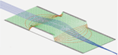SIMION®The field and particle trajectory simulator
Industry standard charged particle optics software
Refine your design: model > simulate > view > analyze > program > optimize it.
News: ASMS 2022 posters. Adaptas IMS will be at ASMS booth 718 for any SIMION needs.

# Child's Law

## Definition

Child's Law (or the Child-Langmuir Law or three-halves-power law) gives the maximum space-charge-limited current in a planar diode of infinite radius (i.e. one-dimensional beam) as a function of the length and potential difference between anode and cathode.

J = K Vd3/2 / d2

where

J = current density (mA mm-2)
Vd = potential difference between anode and cathode (V)
d = distance between anode and cathode (mm)
K is a constant given by K = (4/9) ε0 (-2q/m)1/2 with units mA V-3/2, where
ε0 = permittivity of free-space ~ 8.854187817*10-12 F m-1 [*1]
q/m = charge (C) to mass (kg) ratio of particle (absolute value). For an electron, q/m = 1.758820150*1011 C kg-1[*2]
For an electron, K ~ 0.0023340 mA V-3/2.

Note units on left-side of constant: (F m-1) C1/2 kg-1/2 = (C2 J-1) m-1 C1/2 kg-1/2 = C5/2 J-1 (J-1/2 s-1) = (C s-1) (J/C)-3/2 = A V-3/2.

## Use in CPO Charged Particle Optics Software

The CPO software applies a Child's Law relationship near cathode surfaces in the simulation of space-charge limited thermionic Cathode Emissions and can apply a correction (Langmuir relationship) for non-zero temperature.

## Derivation

Child's Law is a fairly direct result of the Poisson equation:

2 V = - ρ / ε0

where charge density ρ = J/v, for velocity v, and where v can be expressed in terms of kinetic energy and related to potential energy: (1/2) m v2 = -q V, for mass m and charge q. This gives

2 V = dV'/dx = A V-1/2
where A = -J ε0-1 (-(1/2) m/q)1/2 is a constant and V' = dV/dx.

Substitute dx = dV/V' to obtain a separable differential equation and solve, while applying boundary conditions V(x=0) = 0, V(x=d) = Vd, and V'(x=0) = 0:

V' dV' = A V-1/2 dV
V' = dV/dx = 2 A1/2 V1/4
V-1/4 dV = 2 A1/2 dx
(2/3)V3/4 = A1/2 x
where A = (4/9)Vd3/2 d-2 (due to V(x=d) boundary condition)

Now knowing A, we can solve for J in the expression for A:

(4/9)V3/2 d-2 = A = -J ε0-1 (-(1/2) m/q)1/2 implies
J = K V3/2 d-2 where K = (4/9) ε0 (-2q/m)1/2

which is the desired result.

Note also that at x=0, v=0 and ρ=.

## References

Any comments on this web page? (will be sent to Adaptas SIMION Support)